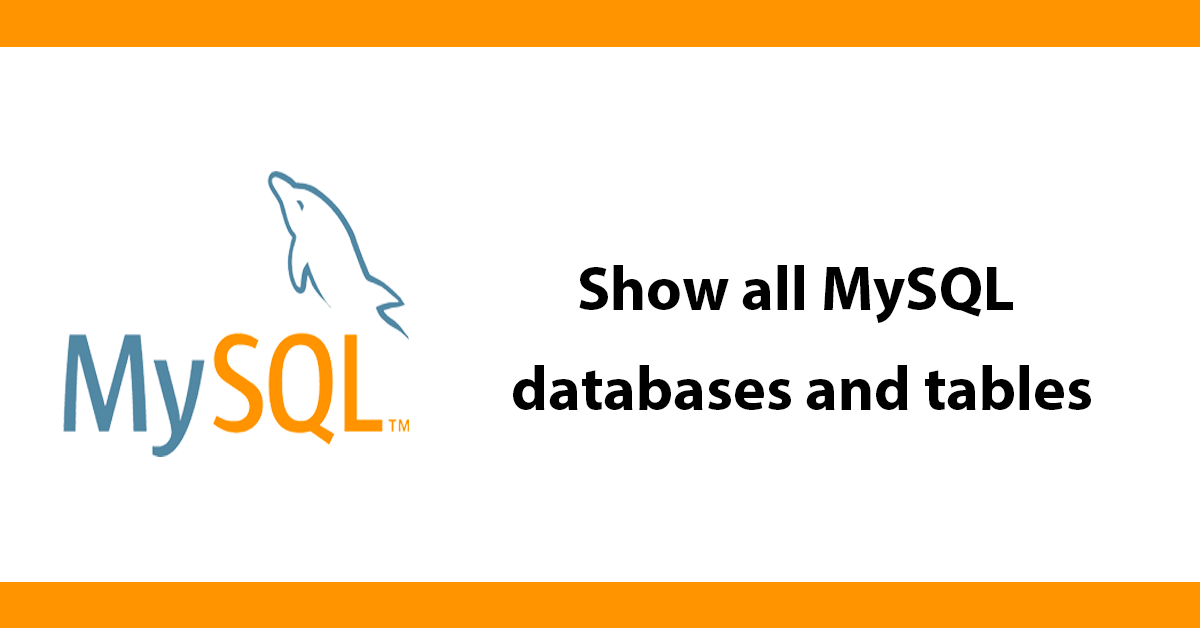# Show all MySQL databases and tables

Want to see what databases you have and what tables are inside each database but do not have access to phpmyadmin or similar program?

Luckily mysql has some built in functions that enable you to see your databases and tables easily.

Let me take you through it.

First make a connection to your database server

``````// connect to database server 'server location', database username', 'password'
\$conn = mysql_connect('localhost','******','*******');``````

Then were going to get all the databases using the function mysql_list_dbs() this function expect a connection parameter to your database once it has that it gets a list of the the databases and stores it in a variable \$dbs then we print out a title for the page then start a unordered list (ul)

``````// list all databases
\$dbs = mysql_list_dbs(\$conn);
echo "databases avaliblen";

echo "<ul>n";``````

Next we need to loop through all the database names we do this using a for loop. First define an variable with a value of 0 \$x=0; then using mysql_num_rows loop while the number of rows is less then the number of databases then increment to complete the loop.

``````// loop through all databases
for(\$x=0; \$x < mysql_num_rows(\$dbs); \$x++)
{``````

To get the names of all databases we use mysql_db_name() with a reference to the mysql_list_dbs by using \$dbs and \$x assign this to a variable then we have a list of all the database names so then we print them out

``````// get name of database
\$db = mysql_db_name(\$dbs, \$x);
echo " <li>" . \$db . "</li>n";``````

Next we get all the tables from the database using mysql_list_tables again using a reference to mysql_db_name and a connection to the database server. Then we start another unordered list

``````//for each db get a list of tables within it
\$tables = mysql_list_tables(\$db, \$conn);
echo "<ul>n";``````

Now we create another for loop to get all the tables, this works the same way the previous for loop worked. The loop will keep looping while the number of tables is less then the variable \$y.

inside the loop echo the list items printing out the table name using mysql_tablename with a reference to the tables and row (\$y)

then close the unordered list, close the for loop and the previous loop and the last unordered list.

``````//get all rows
for(\$y=0; \$y < mysql_num_rows(\$tables); \$y++)
{
echo "    <li>" . mysql_tablename(\$tables, \$y) . "<li>n";
}

echo "</ul>n";
}

echo "</ul>n";``````

Here's the full script:<

``````<?php
// connect to database server
\$conn = mysql_connect('localhost','*******','******');

// list all databases
\$dbs = mysql_list_dbs(\$conn);

echo "databases avaliblen";

echo "<ul>n";

// loop through all databases
for(\$x=0; \$x < mysql_num_rows(\$dbs); \$x++)
{

// get name of database
\$db = mysql_db_name(\$dbs, \$x);

echo " <li>" . \$db . "</li>n";

//for each db get a list of tables within it
\$tables = mysql_list_tables(\$db, \$conn);

echo "<ul>n";

//get all rows
for(\$y=0; \$y < mysql_num_rows(\$tables); \$y++)
{
echo "    <li>" . mysql_tablename(\$tables, \$y) . "<li>n";
}

echo "</ul>n";

}
echo "</ul>n";

?>``````

Now you can see all your tables be running this script in a web browser.Help support the blog so that I can continue creating new content!